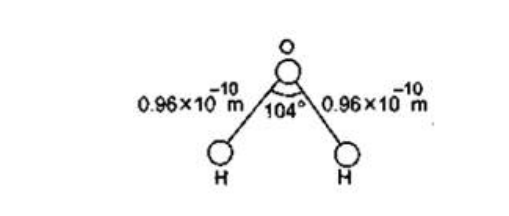Deepak Scored 45->99%ile with Bounce Back Crack Course. You can do it too!

# Solve the following :

Question:

The structure of the water molecule in figure. Find the distance of the center of mass of the molecule from the center of the oxygen atom.Solution:

$\mathrm{O}^{H_{1}}=\alpha=O H_{2}=0.92 \mathrm{~A}^{\circ}$

Take $O^{\prime}$ as oriqin.

then $00^{\prime}=\alpha \cos 52^{\circ}$

$H_{1}=\left(-\alpha \sin 52^{\circ}, 0\right)$

$H_{2}=\left(\alpha \sin 52^{\circ}, 0\right)$

$0=\left(0, \alpha \cos 52^{\circ}\right)=0.59 A^{\circ}$

$x_{C O M}=\frac{m_{H_{1}} x_{H_{1}}+m_{H_{2}} x_{H_{2}}+m_{0} x_{0}}{m_{H_{1}}+m_{H_{2}}+m_{0}}$

$=\frac{\left(-\alpha \sin 52^{\circ} \times 1\right)+\left(+\alpha \sin 52^{\circ} \times 1\right)+(0 \times 16)}{1+1+16}$

$=0$

$y_{C O M}=\frac{m_{H_{1}} y_{H_{1}}+m_{H_{2}} y_{H_{2}}+m_{0} y_{0}}{m_{H_{1}}+m_{H_{2}}+m_{0}}$

$=\frac{0.1+0.1+16 . \alpha \cos 52^{\circ}}{1+1+16}$

$=\frac{16}{18} \times 0.96 A^{\circ} \times \cos 52^{\circ}$

$=0.525 \mathrm{~A}^{\circ}$

Distance from O-atom $=\sqrt{\left(x_{0}-x_{\text {COM }}\right)^{2}+\left(y_{0}-y_{\text {COM }}\right)^{2}}$

$=\sqrt{(0)^{2}+\left(0.59 A^{\circ}-0.525 A^{\circ}\right)^{2}}$

$=0.065 \times 10^{-10} \mathrm{~m}$

$=6.5 \times 10^{-12} \mathrm{~m}$